Magnetostatics - 2

# Magnetostatics - 2 - Notes | Study Electromagnetic Fields Theory (EMFT) - Electrical Engineering (EE)

 1 Crore+ students have signed up on EduRev. Have you?

Magnetic field due to a solenoid and a toroid

A solenoid is essentially a long current loop with closely packed circular turns. The length of the solenoid is very large compared to the diameter of the turns. In diagram shown below, current enters the page from the top and goes behing the page at the bottom. It leads to addition of the magnetic field due to the turns inside the solenoid and cancellation outside.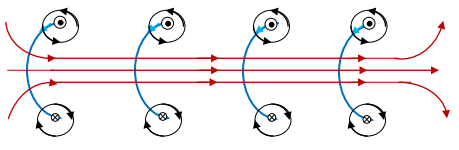The magnetic field can be calculated by Ampere’s law and it gives,

Field inside = μ0nl, where n is the number of turns per unit length of the solenoid. The field outside thec solenoid is zero. The same result can be obtained by use of Biot Savart’s law, which is left as an exercise.

A toroid looks like a doughnut with a central hole and current carrying wires wound over its core. The core is usually made of iron or some such magnetic metal.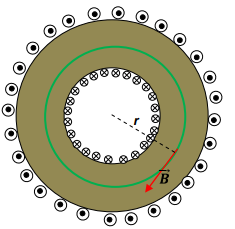Take a circular loop of radius r concentric with the toroid. The amount of current enclosed by the loop is NI where N is the total number of turns and I is the current in each turn. By Ampere’s law, the field is given by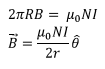where in the last step we have explicitly shown that the field is cuircumferential. The field outside the toroid is zero because the Amperian loop would enclose zero current as current both goes in and comes out through the loop. Likewise, inside the hole the field would also be zero as it would not thread any current. Note that unlike in the case of a solenoid, the field is not uniform.

Force Between Current Loops

We have seen that a magnetic field exerts a force on a moving charge. Since a current loop contains moving charge, it follows that such a loop would experience a force in a magnetic field. Further, since the magnetic field in which a loop experiences a force must have its origin in another current source, two current carrying circuits would exert force on each other.

Consider two such circuits, numbered 1 and 2.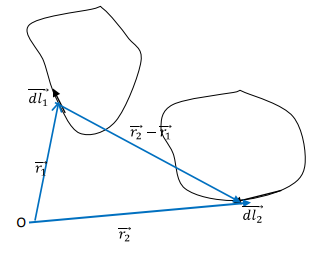Consider the field due to the current element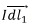in circuit 1. At a position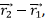the field is given by Biot Savart’s law,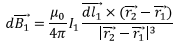The net magnetic field at this point is given by summing over the contribution due to all elemental current comprising the circuit. Since the circuit 2 contains moving charges, the element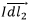experiences a force due to this field which is given by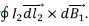By summing over all the current elements in circuit 2, we get the force exerted by circuit 1 on circuit 2 is given by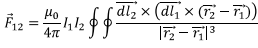This is a very clumsy expression and can be evaluated only in cases of simple geometry.

By symmetry it follows that the force on circuit 1 due to current in circuit 2 is given by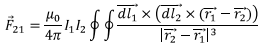It is not obvious from these two expressions that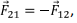as it ought to be from Newton’s third law. We will show that the third law remains valid. This requires a bit of algebraic manipulation. Let us start with the expression for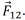The integrand can be written as follows: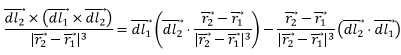Where we have used the vector identity,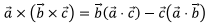As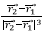is negative gradient of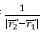with respect to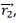we can write the integral over the first term as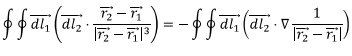If we now perform the second line integral it can be rewritten as a surface integral of the curl of a gradient which is identically zero,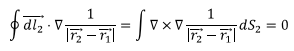We are thus left only with the integral of the second term of the simplified form of integral, which clearly shows the symmetry which we are looking for.

Force between two parallel wires carrying current

Consider two parallel wires carrying current in the same direction. We take both wires in the plane (xy) of the paper. The first wire (to the left) creates a magnetic field which is into the plane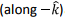at the location of the second wire and is given by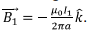The force exerted on a unit length of the second wire is thus given by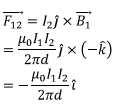Thus the two wires attract each other.

Electric and Magnetic Fields are different manifestations of the same physical phenomenon

We have seen that electric field is produced by static charges whereas the source of a magnetic field is steady current. However, static charge in one reference frame can be seen as moving charges in another reference frame moving with a relative velocity with respect to the first frame. This requires some comments. In the following, we assume familiarity with basic concepts of the special theory of relativity. (For those who are not familiar, this section may be dropped without affecting understanding of subsequent lectures. Such readers should directly go to the section on vector potential in this lecture itself).

If we have a static charge q, it would experience a force on another charge q’, whether or not that charge is moving. The force on the second charge is electrostatic and is given by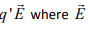is the field at the location of the second charge. If the second charge happens to be moving, the field is the instantaneous field at the , location of the second charge. If we have a steady current, it will create a magnetic field around it which will not exert a force on a static charge though it will exert a sidewise force on a moving charge. This force is given by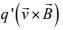and is very much real. Suppose now there is an observer who is moving with the same velocity as that of this charge, i.e. if the observer is in the frame of this moving charge, in the observer’s frame the velocity of the charge being zero, he would not expect any magnetic force . However, both the observers will notice the sidewise deflection of the charge and hence since the force exerted on the charge is not fictitious. How does one resolve this apparent contradiction?

Let us consider the following situation. Suppose we have two, infinite, charged parallel wires carrying linear charge densities λ1 and λ2 separated by a distance d. Let the wires be in the xy plane with their lengths parallel to the x direction.

In a frame S, which is at rest with respect to these wires, these charged wires exert a force on each other (repulsive, if λ1 and λ2 are of the same sign.) We can calculate this force. The electric field due to λ1 at the location of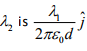where the unit vector is directed from the first wire to the second.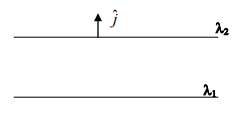If we consider a length L of the second wire (with charge density λ2) the force on the wire (as seen by S) will be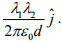Lets us now look at the situation with respect to an observer moving with a speed v along x direction. We know that according this observer, all lengths along x direction are contracted by a factor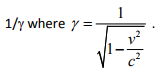Thus in this frame a length L parallel to the x direction is shortened to L/γ.

Note that the charge density increases because the charge itself does not depend on observer and the same charge is now distributed over a shorter length.  Thus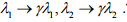The electric force on a section which had a length L in S is seen by S’ to be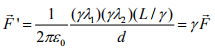Note that the length d being in a transverse direction is not contracted.

This result is not consistent with what we learnt regarding the way a transverse force transforms under Lorentz transformation. Which is given by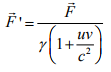where u is the velocity of the object with respect to the moving observer. In this case u=0, so that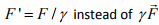calculated by S’.

The anomaly can be removed if S’ invokes the existence of another force in his frame of reference, the magnetic force. Let that force be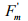so that the total force in S’ frame is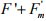which should be equated to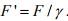Thus S’ invokes a magnetic force given by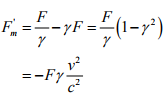Using the expression for F γ,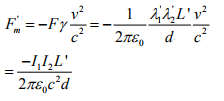the minus sign shows that the force is attractive. This makes perfect sense when viewed from S’ because in his frame the charge densities produce two currents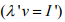which are parallel and hence attract. Thus what was a purely electric force in S becomes partly electric and partly magnetic forces in S’. The observers S and S’ do not agree on origin of the forces but measure the same force.

Conclusion : Electric and magnetic fields are interchangeable, depending on the state of motion of the observer.

Vector Potential

We had seen that the electrostatic field is irrotational (conservative) i.e.,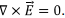This allowed us to introduce a scalar potential φ corresponding to the electric field in terms of which the field is expressed as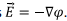This simplified our efforts a lot because a scalar field subject to superposition principle can be easier to deal with.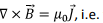i.e. the field is not irrotational. Instead we have the magnetostatic Gauss’s law which gives the field to be solenoidal,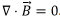This allows us to express the magnetic field as a curl of a vector field because divergence of a curl is identically zero. Thus we define the vector potential corresponding to a magnetic field by the relation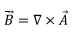We are aware that as per Helmholtz Theorem, a vector potential is uniquely given by specifying its curl and divergence. The curl of the vector potential, being the magnetic field has definite physical meaning. We have the freedom to choose the divergence of the vector potential at our will and usually this is done to make calculations easier. For instance, if we consider the Ampere’s law and express it in terms of the vector potential, we would get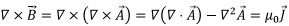We now use our freedom in the choice of the divergence of the vector potential. Since the divergence has no physical significance, let us choose this to be zero. The Ampere’s law then takes the form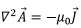We find that each component of the vector potential satisfies a Poisson’s (or Laplace’s) equation. This freedom in choosing the divergence of the vector potential is known as “gauge choice” and the particular choice of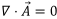is known as the “Coulomb Gauge”.

A question naturally arises does one always have the gauge choice ? Suppose we have calculated a vector potential such that its divergence is non-zero. Since to any such vector potential, we can always add gradient of an arbitrary scalar without affecting the value of the magnetic field (because curl of a gradient is identically zero), let us define a new vector potential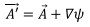where ψ is some scalar field. We then have,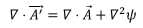Clearly, the new vector potential will satisfy Coulomb gauge condition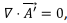if we choose ψ such that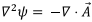This being a Poisson’s equation, a solution can always be found. Thus even if we start with an arbitrary vector potential, we can by suitable transformation, arrive at a new vector potential which satisfies the Coulomb gauge condition.

Let us express the BiotSavart’s law in terms of the vector potential.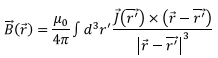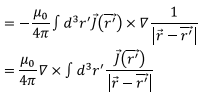In arriving at the last expression we have used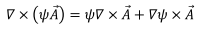In this case the vector field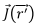is constant in so far as the derivatives with respect to r is concerned so that the first term gives zero. The relative minus sign comes because the order of the cross product is reverse of what we have in the vector identity above. Thus we have,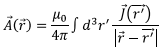Note the similarity of this expression with that of the expression for the potential for the electrostatic field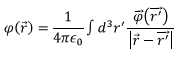The role of charge density is taken by the current density here.

Note that unlike the magnetic field, no useful purpose is served by calculating the vector potential at a single point, one needs to calculate it in a region of space to get an expression for the magnetic field. We will do so in the next lecture.

Tutorial Assignment

1. Two long parallel wires carry charge density λ (in the rest frame of the wires) each, are moving with a velocity v each parallel to their length. Calculate the force per unit length on either of the wires in a reference frame that is (a) at rest in the laboratory and (b) moving along with the wires with a velocity v (i.e. in the rest frame of the moving wires).

2. Two parallel current carrying wires of length L andmass m per unit length each are suspended from the ceiling by means of massless strings of length l each. The wires carry equal currents in opposite directions. If the angle between the wires suspended from the same point is 2θ, obtain an expression for the current flowing in each wire in terms of the given quantities.

Solutions to Tutorial Assignment

1. In a frame that is at rest with the wire (i.e. the observer is moving with the same velocity as the wires) , the only force is electrostatic and the force on either of the wires per unit length is given by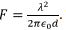The force is repulsive.

For the observer who is at rest in the laboratory, in addition to the electric force, there is also a magnetic force because the moving charge constitutes a current. Since, in this frame (which is moving with a velocity –v with respect to the wires), the lengths are contracted, the charge density is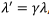the electric force is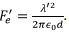The current being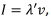the magnetic force between the wires is attractive (parallel currents)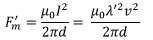The net force is then given by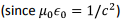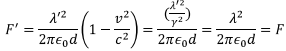2. Let the tension in the strings be T. In equilibrium, we have, T cos θ = mLg, T sin θ = Fm which gives F = mg tan θ.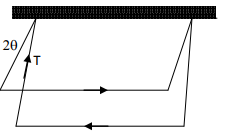The magnetic force is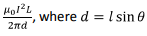is the separation between the wires in equilibrium. Substituting these in the equation F = mg tan θ, the result follows.

Self Assessment Quiz

1. Obtain the expression for the magnetic field on the axis of a finite solenoid carrying n turns per unit length.

2. A rectangular loop of dimension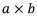carries a current l1. A long wire carrying a current l2 is  is at a distance d  from one of the sides of length a it, the rectangle and the wire are in the same plane. The direction of current in the wire and that flowing in the nearer side of the rectangle are parallel. Calculate the force on the loop

3. A single current loop is in the shape of a right triangle of sides a, b, c. A uniform magnetic field of strength B0 is directed parallel to the hypotenuse. Calculate the total force on the loop as well as the torque acting on the loop.

4. A semicircular loop of radius R lies in the xy plane carrying a current I in the counterclockwise direction. Magnetic field B points perpendicular to the diameter (along the y direction). Calculate the force on the straight-line segment as well as on the semi circular arc.

Solutions to Self Assessment Quiz

1. The turns of the coil are shown below with a dot indicating that the current is coming out of the page and a cross indicating that it is going into the page. Consider a point P on the axis of the solenoid.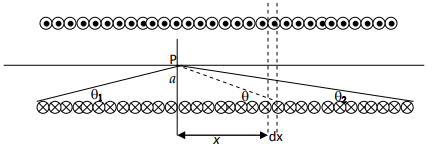Let a be the radius of the solenoid. Consider a length dx at a distance x from P. The amount of current that this section contains is I n (dx). This section can be considered as a circular loop of radius a, the field due to which at P is given by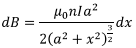Let tan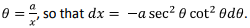The denominator of the above expression is given by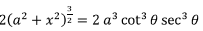The field due to the solenoid at P is given by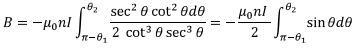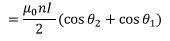As the length of the solenoid approaches infinity both the angles θ1 = θ2 → 0 and we get back B = μ0nl as expected.

2. The force on the two shorter sides cancel because they carry current in the opposite direction and the force on each current element on one of the sides is canceled by the force on the corresponding element on the other side. The force of attraction on the nearer side is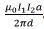and the repulsive force on the far  side is Thus the net force is attractive and is given by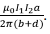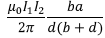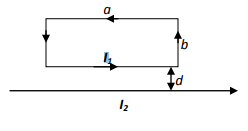3. Let the triangle be in the xy plane. The unit vector along c is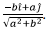Thus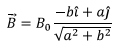Force on side c is zero. Force on side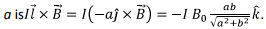It can be shown that the force on the side b is equal and opposite so that the net force is zero. This is also seen by adding up the force vectorially since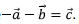The torque is not zero however. As the torque can be written as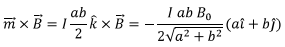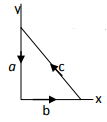4. The force on the straight-line segment is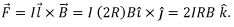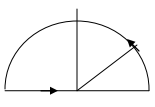Take a length element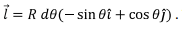The force on the length element is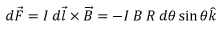Integrating, the force on the arc is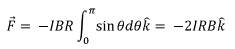(Of course, the net force on the loop has to be zero!

The document Magnetostatics - 2 - Notes | Study Electromagnetic Fields Theory (EMFT) - Electrical Engineering (EE) is a part of the Electrical Engineering (EE) Course Electromagnetic Fields Theory (EMFT).
All you need of Electrical Engineering (EE) at this link: Electrical Engineering (EE)

## Electromagnetic Fields Theory (EMFT)

11 videos|46 docs|62 tests
 Use Code STAYHOME200 and get INR 200 additional OFF

## Electromagnetic Fields Theory (EMFT)

11 videos|46 docs|62 tests

Track your progress, build streaks, highlight & save important lessons and more!

,

,

,

,

,

,

,

,

,

,

,

,

,

,

,

,

,

,

,

,

,

;